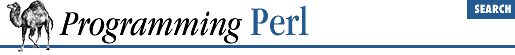home | O'Reilly's CD bookshelfs | FreeBSD | Linux | Cisco | Cisco Exam

#### 3.2.154 splice

```splice ```

ARRAY
```
, ```

OFFSET
```
, ```

LENGTH
```
, ```

LIST
```

splice ```

ARRAY
```
, ```

OFFSET
```
, ```

LENGTH
```

splice ```

ARRAY
```
, ```

OFFSET
```
```

This function removes the elements designated by ``` OFFSET ``` and ``` LENGTH ``` from an array, and replaces them with the elements of ``` LIST ``` , if any. The function returns the elements removed from the array. The array grows or shrinks as necessary. If ``` LENGTH ``` is omitted, the function removes everything from ``` OFFSET ``` onward. The following equivalences hold (assuming \$[ is 0):

Direct Method Splice Equivalent
``` push(@a, \$x, \$y)``` ``` splice(@a, \$#a+1, 0, \$x, \$y)```
``` pop(@a)``` ``` splice(@a, -1)```
``` shift(@a)``` ``` splice(@a, 0, 1)```
``` unshift(@a, \$x, \$y)``` ``` splice(@a, 0, 0, \$x, \$y)```
``` \$a[\$x] = \$y``` ``` splice(@a, \$x, 1, \$y);```

The splice function is also handy for carving up the argument list passed to a subroutine. For example, assuming list lengths are passed before lists:

```sub list_eq {       # compare two list values
my @a = splice(@_, 0, shift);
my @b = splice(@_, 0, shift);
return 0 unless @a == @b;       # same len?
while (@a) {
return 0 if pop(@a) ne pop(@b);
}
return 1;
}
if (list_eq(\$len, @foo[1..\$len], scalar(@bar), @bar)) { ... }```

It would probably be cleaner just to use references for this, however.